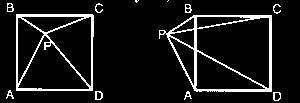##Given a square ABCD and a point P in the plane (either picture above is correct), find PD and AB in the problems below.

Problem 1: (PA, PB, PC) = (4, 3, sqrt(10) )
Problem 2: (PA, PB, PC) = (7, 3, 9)

For each problem, there is only one answer for PD. There are two answers for AB, corresponding to the point P being inside or outside of the square. Finding PD is easier than finding AB.

Source: 1. Internet newsgroup rec.puzzles, 2. Original
[Taken from "Ken's Puzzle of the Day" February 24, 1994]
Solution